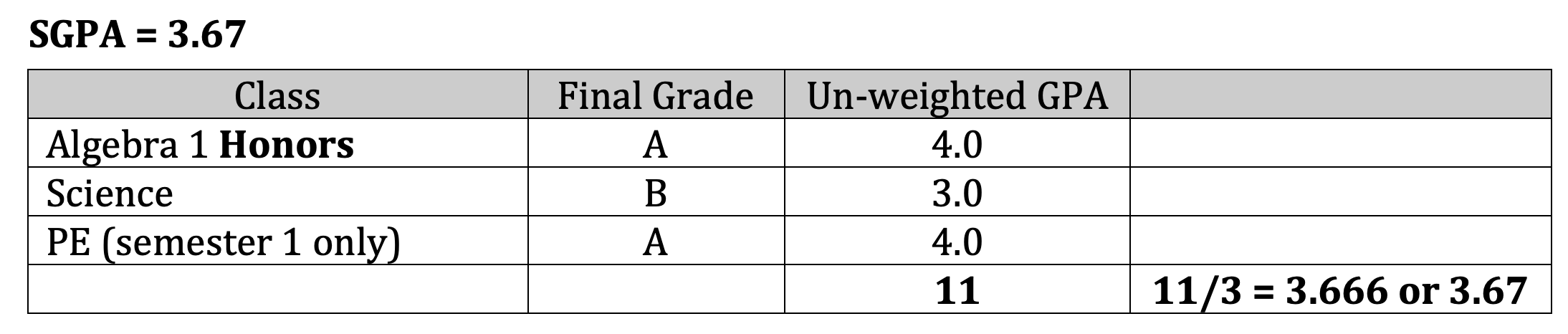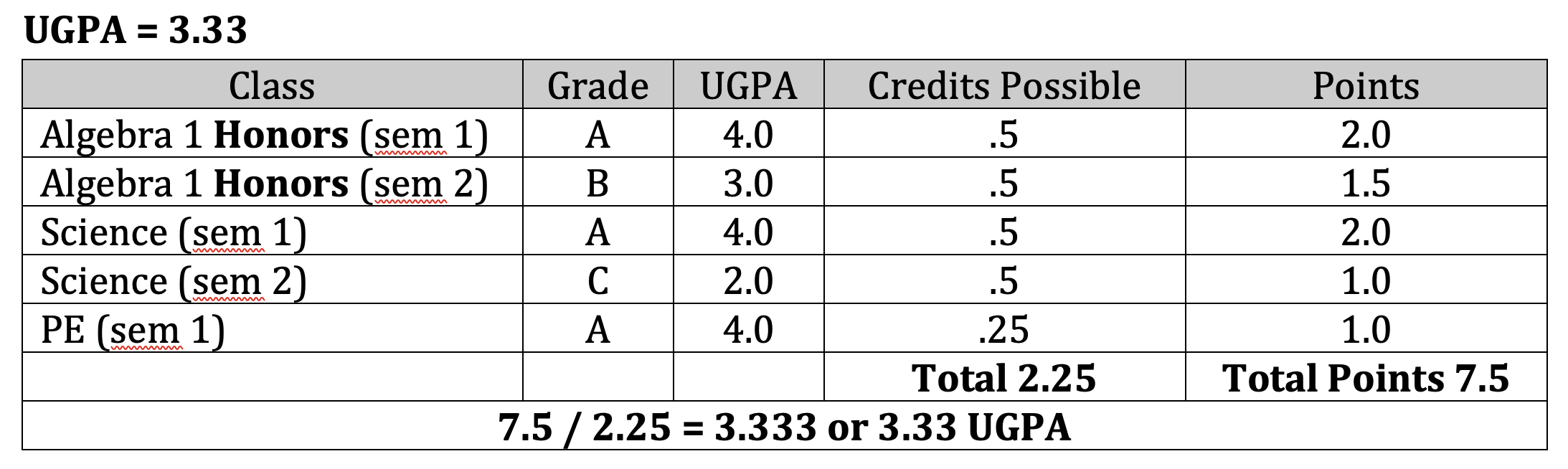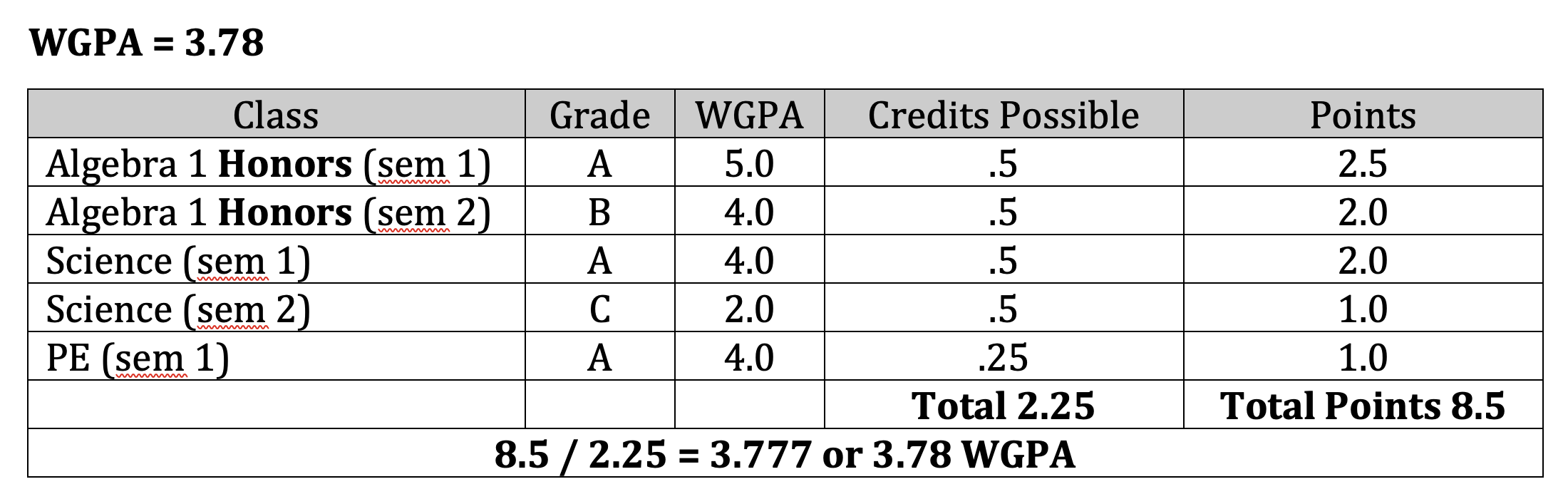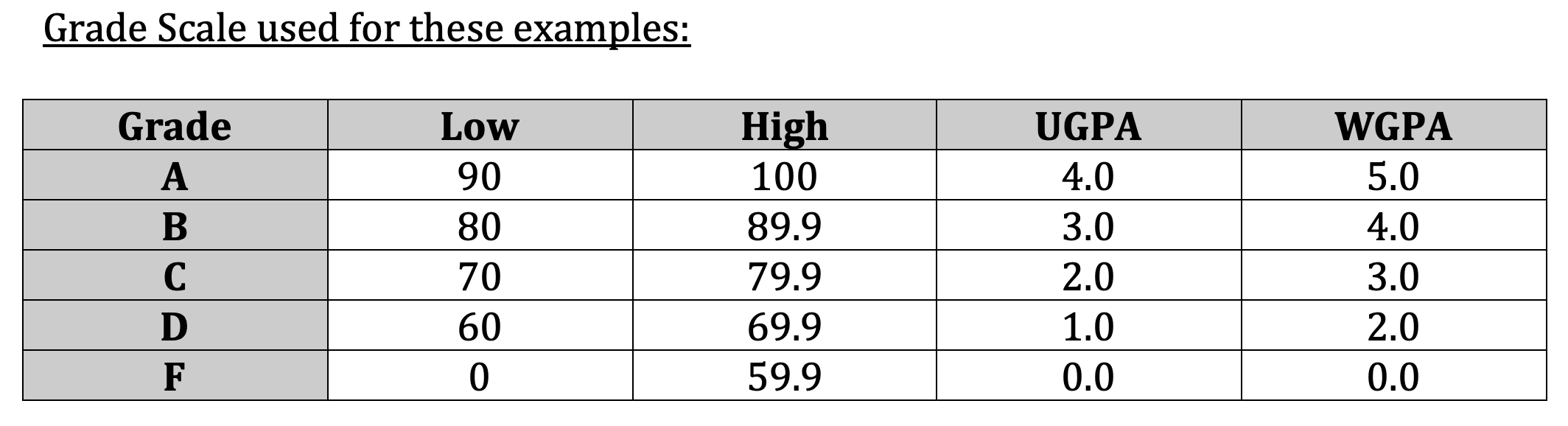# GPA Explanations

## What is the difference between SGPA, UGPA, and WGPA?

#### 1. SGPA - Simple GPA

The SGPA is calculated by using the unweighted GPA points for the final grade for each subject. These GPA points are added together and then divided by the total number of classes taken to get the SGPA. Each class is included in the total number of classes regardless of how many semesters a class took place. (example: PE only took place during the first semester.)#### 2. UGPA - Unweighted GPA

The UGPA is calculated using semester grades. The unweighted GPA for each subject's semester grade is multiplied by the possible credits for that subject for that semester and gives us a number of points for that subject for that semester. These quality points are added together and then divided by the toal credits possible during the time period included in the calculation. This gives the UGPA or unweighted GPA.#### 3. WGPA - Weighted GPA

*Honors classes, for this example, were given 1 full point extra. (A = 5.0, B = 4.0, C = 3.0...)*

The WGPA earned by each subject's semester grade is multiplied by the possible credits for that semester to give the points earned for each class per semester. The points are added together and the possible credits are added together to give the totals for each for an academic year or for all academic years combined, if multiple years are involved. Total points divided by total possible credits equals WGPA.